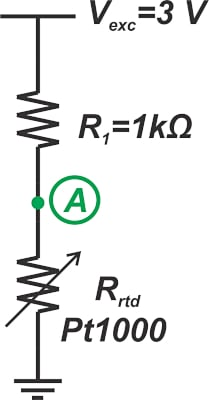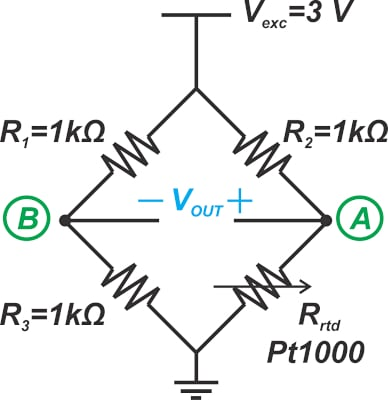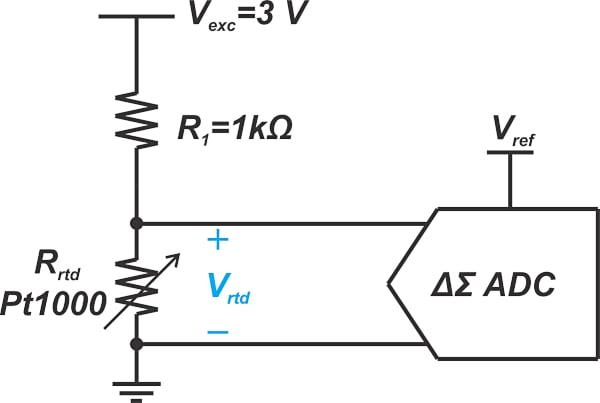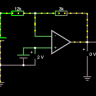Technical Article

# An Introduction to RTD Sensor Signal Conditioning Circuits

October 30, 2022 by Dr. Steve Arar

## Learn about the basics of different signal conditioning circuits for RTD applications, including a voltage divider, Wheatstone bridge circuit, and delta-sigma (ΔΣ) converter.

In previous articles in this series, we discussed the basic principles of resistance temperature detectors (RTD) and how their response is characterized. This article will discuss the basics of different signal conditioning circuits for RTD applications.

### Using a Voltage Divider for RTD Measurement

A simple resistive voltage divider can be used to convert the variations in the RTD resistance to a voltage signal. Figure 1 shows a typical circuit diagram for a platinum RTD. The Pt1000 in the figure denotes a platinum RTD with a nominal resistance of 1000 Ω at 0 °C.##### Figure 1. Example circuit diagram for a platinum RTD.

Like most resistive sensors, RTD sensors change by a relatively small percentage in response to variations in the measured physical quantity. With that in mind, Pt1000 has a temperature coefficient of about 3.85 Ω/°C. Let’s see how large the voltage variations at node A are.

Assume that we need to measure temperature by a resolution of 0.2 °C, which can be a relatively demanding requirement. If the temperature changes from 0 °C to 0.2 °C, the sensor resistance increases from 1000 Ω to 1000.77 Ω. This consequently changes the voltage of node A from 1.5 V to 1.500577 V, as calculated below:

$V_{A}=\frac{R_{rtd}}{R_{rtd}+R_{1}}\times V_{exc}=\frac{1000.77\times3}{1000.77+1000}=1.500577V$

Therefore, changing temperature by 0.2 °C changes the voltage of node A by about 577 μV. We can directly measure VA to determine the RTD resistance value and temperature; however, our measurement system should have enough resolution to detect fraction-of-a-millivolt variations in a 1.5V signal. Dividing 1.5 V to the minimum required step size (577 μV), we can estimate the noise-free counts of the analog-to-digital converter, which works out to:

$Noise\,Free\,Counts=\frac{1.5V}{577 \mu V}\approx2600\,counts$

This corresponds to a noise-free resolution of about log2(2600) = 11.34 bits. Note that this only gives us an approximate value of the A/D resolution. The actual requirement is more stringent and depends on the temperature range for which the thermometer is designed. Also, we modeled the RTD with a constant temperature coefficient of 3.85 Ω/°C, whereas RTDs are actually non-linear devices.

A noise-free resolution of 11 bits can be easily achieved by today's delta-sigma (ΔΣ) converters. Thus, we can use the circuit in Figure 1, along with a ΔΣ converter, to directly digitize the voltage across the RTD.

Decades ago, however, such high-performance data converters were not available or economical; and circuit designers used techniques such as Wheatstone bridge circuits for RTD measurements. While bridge circuits are still commonly used in other areas, such as force and pressure sensing applications, they are seldom used for RTD measurements. Despite that, for the sake of completeness, we’ll briefly discuss below how a bridge circuit can relax the analog-to-digital converter (ADC) requirements.

### The Traditional Method: Using a Wheatstone Bridge for Pt1000 Measurement

A basic Wheatstone bridge for Pt1000 measurement is shown in Figure 2.##### Figure 2. An example of a Wheatstone bridge measurement for Pt1000.

The output voltage is the voltage difference between the two branches. In fact, a bridge circuit changes the single-ended measurement from a simple voltage divider branch to a differential measurement. In this case, the output is 0 V when the bridge is balanced (at 0 °C). If the temperature increases by 0.2 °C, the output increases to 577 μV  as calculated below:

$V_{OUT}=V_{A}-V_{B}=\frac{1000.77\times3}{1000.77+1000}-\frac{1000\times3}{1000+1000}=577\mu V$

In this case, the desired signal that reflects the RTD resistance variations is not riding on top of a large DC signal. The output contains only the signal that we want to measure. To determine the noise-free resolution of the ADC, we should consider the maximum and minimum values of VOUT over the entire temperature range of the thermometer. Assume that we need to measure the -40 °C to 150 °C range. The RTD resistance changes from 842.47 Ω to 1573.25 Ω over this temperature range. We can use this information to determine the maximum and minimum value of VOUT as calculated in Table 1 below:

##### Table 1.
Temperature (°C) Rrtd (Ω) VOUT (V)
-40 842.47 -0.128249
150 1573.25 0.334159

Since the minimum change that should be detected is 577 μV, the noise-free counts of the system can be calculated by:

$Noise\,Free\,Counts\,=\frac{V_{OUT,max}-V_{OUT,min}}{Step\,Size}=\frac{0.334159-(-0.128249)}{577\mu V}\approx 802\,counts$

Which corresponds to a noise-free resolution of 9.65 bits. As you can see, the ADC resolution obtained for the entire 190 °C temperature range of the bridge-based measurement is still more relaxed than that obtained for a single measurement of the voltage divider approach.

#### Bridge Circuit Limitations for RTD Applications

Although bridge circuits can ease the ADC requirements, this method has a few disadvantages. The bridge output is dependent on the value of the resistors employed in the bridge configuration. This limitation is why three precision resistors are needed to complete the bridge. Besides that consideration, bridges with a single sensing element are nonlinear. Therefore, in addition to the RTD nonlinearity, the designer must compensate for the bridge’s nonlinear response. Software or analog techniques can be used to linearize bridge circuits, adding to the system's complexity. When using bridge circuits, we also need instrumentation amplifiers with large common-mode rejections that can provide high and equal input impedances.

Due to these limitations and noting that modern delta-sigma converters can easily meet and defeat the requirements of RTD applications, circuit designers don’t usually use bridge circuits for RTD measurement.

### RTD Sensor Measurement Using a Delta-sigma Converter

Figure 3 shows a simplified diagram for interfacing an RTD sensor with a ΔΣ ADC.##### Figure 3. A simplified diagram for interfacing with an RTD sensor and a ΔΣ ADC

With a 22-bit ADC and a reference voltage of 3 V, the LSB (least significant bit) is equal to $$\frac{3}{2^{22}}\approx0.72\,\mu V$$.

With these high-resolution ADCs, the minimum detectable signal is typically limited by the electronic noise within the ADC, e.g. the thermal noise and flicker noise generated by the internal circuitry, rather than the quantization noise of the ADC. If you need to brush up on the noise performance of ΔΣ ADCs, you can refer to this excellent twelve-part article series from Texas Instruments.

The peak-to-peak input-referred noise of a ΔΣ ADC can be in the order of microvolts or lower. Let’s assume that the ADC has an input-referred noise of 3 μVp-p. For the circuit in Figure 3, we can find the maximum and minimum value of the RTD voltage, Vrtd, as calculated in Table 2 below:

##### Table 2.
Temperature (°C) Rrtd (Ω) Vrtd (V)
-40 842.47 1.3717
150 1573.25 1.8342

Using this information, we can calculate the noise-free counts of the system over the -40 °C to 150 °C temperature range as follows:

$Noise\,Free\,Counts\,=\frac{V_{OUT,max}-V_{OUT,min}}{Input-Referred\,Noise}=\frac{1.8342 - 1.3717}{3\mu V}=154166\,counts$

Dividing the temperature range by the noise-free counts gives us the temperature measurement resolution:

$Temperature Resolution=\frac{T_{max}-T_{min}}{Noise\,Free\,Counts}=\frac{150-(-40)}{154166}=0.0012°C$

While this level of precision is actually thrilling, it should be noted that several other error sources prevent us from achieving such a high performance. The initial tolerance and temperature drift of R1 and the ADC offset voltage and offset drift are a few of these error sources. The above calculations, however, confirm that the noise performance and resolution of modern ADCs are sufficient for precision thermometry; however, the designer needs to eliminate other primary error contributors to maintain system accuracy.

Note that in the above examples, a relatively small value is chosen for the bias resistor R1. In practice, a larger resistor might be needed to limit the RTD self-heating effect.

### Ratiometric Measurement for RTD Applications

While different diagrams in this article use a voltage source to excite the RTD, many RTD applications use a current source for sensor excitation. Also, RTD applications commonly derive the ADC reference voltage from the same source that excites the sensor. This technique, known as ratiometric measurement, minimizes errors caused by undesired variations in the sensor excitation source or the ADC voltage reference. In the next article, we’ll continue this discussion and see how RTD applications can benefit from ratiometric measurements.•Dan.K February 27, 2023

Text and calculation differ: “This consequently changes the voltage of node A from 1.5 V to 1.50288 V, as calculated below:” but the calculation results in 1.500577. Just a typo or what do I miss here?
V.

Like.
• K
Kimber Wymore February 27, 2023
Hello! Thank you for bringing this error to our attention. We've since corrected it. We appreciate your help keeping our articles accurate!
Like.
• D
Dr. Steve Arar February 27, 2023
Thank you for pointing this out! It was a typo. The correct value is 1.500577 V.
Like.